• 离散数学——真值表判断
千次阅读
2020-04-13 23:25:59

离散数学_真值表判断

算法小白
代码比较冗长
主要使用了栈和一个二进制转换

对于题目来说，需要解决的地方应该是括号的优先级
大佬都晓得，栈是解决优先级的好办法

所以
二话不说，上代码
(C++写的)


cpp
#include<cstdio>
#include<iostream>
#include<cstring>
#include<string>
#include<cctype>
#include<stack>
#include<algorithm>
using namespace std;
char m;//字母名称
stack<char>s, o;
string change(int n)
{
string str1;
int cnt = 0;
string ans;
while (n)
{
str1 += n % 2 + '0';
n = n / 2;
}
string re;
if (str1 == re)
{
str1 = '0';
}
reverse(str1.begin(), str1.end());
return str1;
}//二进制转换
const char and_cal(char a, char b)
{
if (a == '1' && b == '1')return '1';
else return '0';
}
const char or_cal(char a, char b)
{
if (a == '1' || b == '1')
return '1';
else return '0';
}
const char two_cal(char a, char b)
{
if ((a == '1' && b == '1') || (a == '0' && b == '0'))return '1';
else return '0';
}
const char no_cal(char a)
{
if (a == '0')return '1';
else return '0';
}
const char one_cal(char a, char b)
{
if (a == '1' && b == '0')return '0';
else return '1';
}//5个运算规则

int main()
{
cout << "\t真值表判断\n";
cout << "规则：\n";
cout << "输入公式要由（ ）确定优先级\n";
cout << "(1) 用 ! 代替 非 操作\n(2) 用 && 代替 ∧ 操作\n(3) 用 || 代替 ∨ 操作\n(4) 用 <> 代替 双条件 操作\n(5) 用 - 代替 → 操作\n";
string str;
string strre;//复制str在以后的每次改变中起到复原作用
int cnt = 0;
cin >> str;
strre = str;
int len = str.length();
for (int i = 0; i < len; i++)
{
if (isalpha(str[i]))
{
for (int j = 0; j < cnt; j++)
{
if (str[i] == m[j])
continue;
}
m[cnt++] = str[i];//字母种类
}

}//找到字母
sort(m, m + cnt);
for (int i = 0; i < cnt; i++)
printf("%c\t", m[i]);
cout << str << "\n";
int d = pow(2, cnt);
for (int a = 0; a < d; a++)
{
str = strre;//每一次替换都会改变原式，此处恢复原式
while (!s.empty())s.pop();
int go = 0;
string num = change(a);//num为二进制表
int aa = cnt;
for (int i = 0; i < cnt; i++)
{
char rr = m[i];//记录当前准备替换的字母
int re = num.length() - aa;
if (re < 0)
{
cout << "0\t";
for (int d = 0; d < str.length(); d++)
if (str[d] == rr)str[d] = '0';
}//二进制前导0处理
else
{
char nn = num[num.length() - aa];
cout << nn << "\t";
for (int d = 0; d < str.length(); d++)
if (str[d] == rr)str[d] = nn;
}
aa--;
}//字母替换
for (int i = 0; i < len; i++)
{
s.push(str[i]);
if (str[i] == ')')
{
s.pop();//弹出右括号
while (!o.empty())o.pop();
while (s.top() != '(')
{
o.push(s.top());
s.pop();
}
s.pop();//弹出前括号

while (o.size() != 0)
{
char k = o.top();
o.pop();
if (o.top() == '&')
{
o.pop();
o.pop();
char c = and_cal(k, o.top());
s.push(c);
o.pop();
}
else if (o.top() == '|')
{
o.pop();
o.pop();
char c = or_cal(k, o.top());
o.pop();
s.push(c);
}
else if (o.top() == '<')
{
o.pop();
o.pop();
char c = two_cal(k, o.top());
o.pop();
s.push(c);
}
else if (k == '!')
{
char c = no_cal(o.top());
s.push(c);
o.pop();
}
else if (o.top() == '-')
{
o.pop();
char c = one_cal(k, o.top());
o.pop();
s.push(c);
}
}

}
}//去除括号
while (s.size() != 0)
{
if (s.size() == 1)
{
cout << s.top() << endl;
break;
}
char k = s.top();
s.pop();
if (s.top() == '&')
{
s.pop();
s.pop();
char c = and_cal(k, s.top());
s.pop();
s.push(c);
}
else if (s.top() == '|')
{
s.pop();
s.pop();
char c = or_cal(k, s.top());
s.pop();
s.push(c);
}
else if (s.top() == '>' || s.top() == '<')
{
s.pop();
s.pop();
char c = two_cal(k, s.top());
s.pop();
s.push(c);
}
else if (s.top() == '!')
{
s.pop();
char c = no_cal(k);
s.push(c);
}
else if (s.top() == '-')
{
s.pop();
char c = one_cal(s.top(), k);
s.pop();
s.push(c);
}
}
}
}

上一张代码运行的图片(vs2019环境)
样例：((p&&Q)||R)<>(!S)
枯辽，图片只能通过链接去访问。。（有木有大佬知道怎么办emmm）
如果有小伙伴发现代码的bug欢迎骚扰，小菜鸡会在第一时间改正！c++
更多相关内容
• 南京邮电大学实验真值表法求主析取范式和主合取范式代码 离散数学 实 验 一利用真值表法求取主析取范式以及主合取范式的实现 实验名称：利用真值表法求取主析取范式以及主合取范式的实现 实验目的：通过编程实现主...
• 一、实验目的 熟悉五个真值表，掌握真值表...本实验要求从键盘输入一个命题公式列出其真值表。用C语言或MATLAB实现。 三、实验源程序及结果截图 1.实验源程序： #include <stdio.h> #include <stdlib.h>

一、实验目的

熟悉五个真值表，掌握真值表技术。

二、实验内容

定义1 设命题变元P1、P2、P3、…、Pn是出现在公式G中的所有命题变元，指定P1、P2、P3、…、Pn 的一组真值，则这组真值称为G的一个解释或指派，常记为Ｉ。
定义2 真值表：公式G在其所有可能的解释下所取真值的表。
本实验要求从键盘输入一个命题公式列出其真值表。用C语言或MATLAB实现。


三、实验源程序及结果截图
1.实验源程序：

#include <stdio.h>
#include <stdlib.h>
#include <string.h>
int a,b;
int split(char* s)
{
//只有一个数据
if(s==0)
switch(s)
{
case 'a':return a;
case 'b':return b;
}
//判断第一个符号是否为左括号
int i=0;
int l,r;
if(s!='(')//如果不是，用l暂存数据
{
switch(s)
{
case 'a':l=a;
break;
case 'b':l=b;
break;
}
i++;
}
else//如果是左括号，将左括号之后及对应的右括号之前的字符串暂存到s1中
{
i++;
char s1;
int j;
for(j=0;s[i]!=')';j++,i++)
s1[j]=s[i];
l=split(s1);//递归调用，使符号前面的数据为l
printf("%c",l);
}
char t;
int j,k;
for(j=0,k=i+1;s[k]!=0;k++)
{
if(s[k]!='('&&s[k]!=')')t[j++]=s[k];
}
t[j]=0;
r=split(t);//递归调用，使符号后面的数据为r
switch(s[i])
{
case '&':{//合取运算
if(l==1&&r==1)
return 1;
else
return 0;
}
case '|':{//析取运算
if(l==0&&r==0)
return 0;
else
return 1;
}
case '*':{//单条件运算
if(l==1&&r==0)
return 0;
else
return 1;
}
case '+':{//双条件运算
if(l==r)
return 1;
else
return 0;
}
}
}
int main() {
char s;
printf("仅支持含有两个变元的复合命题（分别用a、b表示）\n");
printf("假定运算符优先级相同，输入复合命题时将联结词两端的子公式用（）括起来\n");
printf("合取符号：&   析取符号： |   单条件符号：*   双条件符号：+    不支持否定\n");
printf("请输入命题公式:\n");
scanf("%s",&s);
printf("真值表为：\n");
printf("a\tb\t%s\n",s);
a=1;
b=1;
printf("1\t1\t%d\n",split(s));
a=1;
b=0;
printf("1\t0\t%d\n",split(s));
a=0;
b=1;
printf("0\t1\t%d\n",split(s));
a=0;
b=0;
printf("0\t0\t%d\n",split(s));
return 0;
}

展开全文• 我们介绍了什么是合式公式以及怎样用C语言实现判断机制现在我们来谈谈怎样用C语言去实现合式公式的真值表。 该程序功能就是任意给定一个合式公式我们都能把它的真值表输出出来。步骤1： 引用上一篇的程序，你给我一...

我们介绍了什么是合式公式以及怎样用C语言实现判断机制

现在我们来谈谈怎样用C语言去实现合式公式的真值表。该程序功能就是任意给定一个合式公式我们都能把它的真值表输出出来。

步骤1： 引用上一篇的程序，你给我一个公式首先我得知道它是不是合式公式，这就用到了判断一个公式是否合式公式的方法(详情参照我的上一篇博客)

步骤2： 经过步骤1的检查，我们知道该公式是合式公式，那么就要求真值表了。求真值表本质上也是一个递归的过程，见下图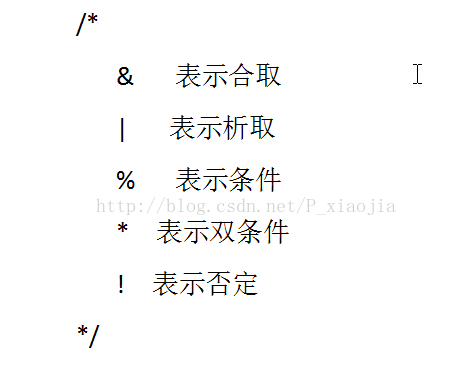步骤3：我们知道了真值表也是个递归的过程，但是合式公式本身只是一串字符无法进行0和1的运算，我们可以让它带上值

利用一下结构体

typedef struct //储存变量带值

{

char ch[Object] ;//储存变量名

bool value[Object] ;//用来放0和1

}Val ;

这样我们就可以把每个变量进行带值运算了 ，当然这里还要把变量从合式公式中抽取出来在放到结构体中。

步骤4：计算变量个数，将变量赋值到结构体数组中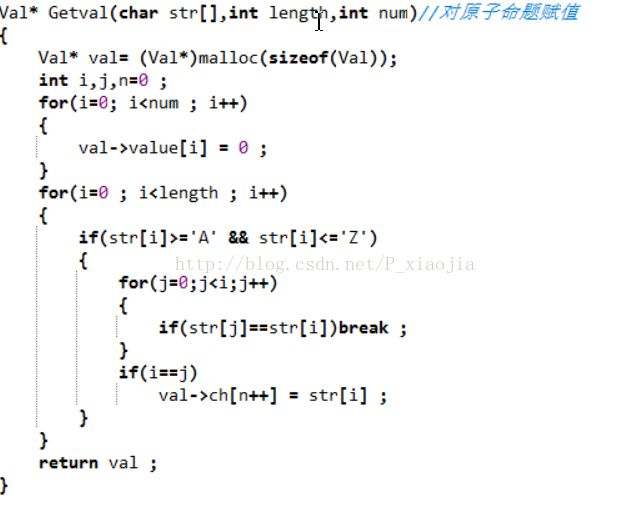步骤5  二级制赋值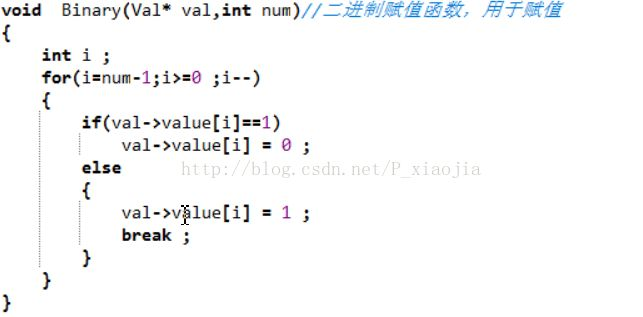步骤6：按分解过程进行计算

最终效果如下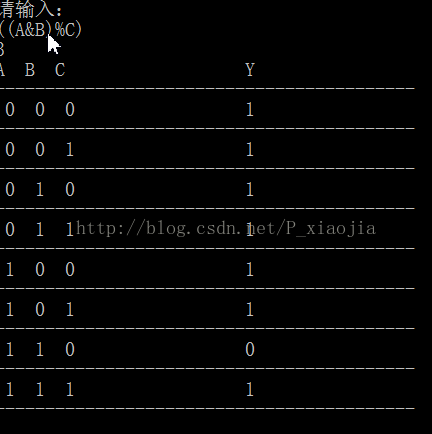展开全文• 离散数学 真值表

# 灵感来源——《栈的应用——四则运算表达式求值》

这个网上文章怪多的，就不赘述了。

博客园 ：Been_You1997CSDN：宝宝丶0807
https://www.cnblogs.com/didideblog/p/7096396.htmlhttps://blog.csdn.net/lub0807/article/details/37884417

# 代码如下

已对代码进行尽可能的注释，希望对你有帮助

因为本人才疏学浅，若有错误及不足之处，欢迎指正
#include<iostream>
#include<string>

using namespace std;

class TrueValueTable
{
private:
char str = { '\0' };// 输入的字符的备份
char str2 = { '\0' };// 后缀表达式
char strOfStack = { '\0' };// 栈数组
char proposition = { '\0' };// 命题元素
int sizeOfStr = 0;
int sizeOfStr2 = 0;
int sizeOfStack = 0;
int sizeOfProposition = 0;
int priority = 5; // 栈顶元素的优先值
bool trueOrFalse = { 0 };// 命题元素的真假值

public:
// 输入字符串，并制作备份
TrueValueTable(const string& strIn)
{
// 将输入的字符串，拷贝到类中，避免更改原字符串后影响后续工作
for (int i = 0; i < 40 && i < strIn.length(); i++)
{
str[i] = strIn[i];
sizeOfStr++;
}
GetProposition();
}

// 获取命题元素
void GetProposition()
{
bool have = 0;// 用来保存遍历数组时，是否存在同名的命题元素名称
for (int i = 0; i < sizeOfStr; i++)
{
have = 0;
if (str[i] >= 'a' && str[i] <= 'z')
{
// 遍历数组，确定字符串中的命题元素是否已经保存至数组proposition
for (int j = 0; j < sizeOfProposition; j++)
if (str[i] == proposition[j])
have = 1;
// 若不存在，则保存至数组中
if (!have)
proposition[sizeOfProposition++] = str[i];
// 此处可为了命题元素有序，进行一此命题元素（a,b,c,d....)的排序
// 命题元素的顺序，不会影响到其表达式的计算
// 除写排序算法外不需做任何更改
/*此处*/;
}
}
}

// 将中缀表达式转换为后缀表达式
void InfixTransSuffix()
{
// 中缀表达式转后缀表达式这个你们自己参考文章
// 自认为写的不好 -_- 抱歉
for (int i = 0; i < sizeOfStr;)
if (str[i] <= 'z' && str[i] >= 'a')
str2[sizeOfStr2++] = str[i++];
else
switch (str[i])
{
case '!':
if (str[i + 1] != '(' && str[i+1]!='!')
{
str2[sizeOfStr2++] = str[i + 1];
str2[sizeOfStr2++] = str[i++];
i++;
}
else
StackPush(str[i++]);
priority = GetPriority();
break;

case '*':
StackPush(str[i++]);
priority = GetPriority();
break;

case '+':
if (priority < 2)
while (StackTop() != '(' && StackTop())
str2[sizeOfStr2++] = StackPop();
else
StackPush(str[i++]);
priority = GetPriority();
break;

case '-':
if (priority < 3)
while (StackTop() != '(' && StackTop())
str2[sizeOfStr2++] = StackPop();
else
StackPush(str[i++]);
priority = GetPriority();
break;

case '=':
if (priority < 4)
while (StackTop() != '(' && StackTop())
str2[sizeOfStr2++] = StackPop();
else
StackPush(str[i++]);
priority = GetPriority();
break;

case '(':
StackPush(str[i++]);
priority = GetPriority();
break;
case ')':
while (StackTop() != '(' && StackTop())
str2[sizeOfStr2++] = StackPop();
StackPop();
if (StackTop() == '!')
str2[sizeOfStr2++] = StackPop();
i++;
priority = GetPriority();
break;
default:
i++;
break;
}
while (StackTop())
str2[sizeOfStr2++] = StackPop();
return;
}

// 输出真值表
void OutputTrueValueTable()
{
/* 首先输出一排命题元素名称（a,b,c...）
以便使用人员能够确定命题元素所对应的值
*/
for (int i = 0; i < sizeOfProposition; i++)
cout << proposition[i] << " ";
cout << '\t' << str << endl;
/*
pow(2,sizeOfProposition)：
计算有sizeOfProposition个命题元素时，
其真值组合的数量，
以便输出足够数量的真值组合
*/
for (int j = 0; j < pow(2, sizeOfProposition); j++)
{
// 输出此时命题元素的真值组合
for (int i = 0; i < sizeOfProposition; i++)
cout << trueOrFalse[i] << " ";
// 进行类似《栈的应用——四则运算表达式求值》
// 方式的后缀表达式的计算
for (int i = 0; i < sizeOfStr2; i++)
{
if (str2[i] >= 'a' && str2[i] <= 'z')
StackPush(str2[i]);
else
switch (str2[i])
{
case '!':
Non();
break;
case '*':
And();
break;
case '+':
Or();
break;
case '-':
Condition();
break;
case '=':
DCondition();
break;
default:
break;
}
}
// 将计算得到的真值输出
cout <<'\t'<< (StackPop() == 0 ? "False" : "True") << endl;
// 使命题元素的真值组合规律的发生变化
// 类似于二进制的自加运算
ChangeValueOfProposition();
}
}

// 确定栈顶元素的优先值
int GetPriority()
{
/*
第二遍使用栈时，栈主要用来存储运算符
因此栈顶有可能是运算符，也有可能是‘（’
需要排除‘（’的影响
*/
if (StackTop() == '(')
switch (strOfStack[sizeOfStack - 1])
{
case '*':
return 1;
break;
case '+':
return 2;
break;
case '-':
return 3;
break;
case '=':
return 4;
break;
default:
return 5;
break;
}
else
switch (strOfStack[sizeOfStack - 1])
{
case '*':
return 1;
break;
case '+':
return 2;
break;
case '-':
return 3;
break;
case '=':
return 4;
break;
default:
return 5;
break;
}
}

// 栈压入数据
void StackPush(const char& charIn)
{
// 往栈顶添加元素后，使栈的长度加一
strOfStack[sizeOfStack++] = charIn;
}

// 栈弹出数据
char StackPop()
{
// 如果栈的长度不为零，则返回栈顶元素，并使栈的长度减一
return sizeOfStack != 0 ? strOfStack[--sizeOfStack] : 0;
}

// 返回栈顶元素
char StackTop()
{
// 如果栈的长度不为零，则返回栈顶元素
return sizeOfStack == 0 ? 0 : strOfStack[sizeOfStack - 1];
}

// 获取命题的值
bool GetValueOfProposition(const char& proposi)
{
// 遍历命题元素数组，若找到同名命题，则返回对应的真值
for (int i = 0; i < sizeOfProposition; i++)
if (proposition[i] == proposi)
return trueOrFalse[i];
// 若没找到同名命题，则返回传入的参数
// 一般，未找到命题元素，代表这个值应为0 / 1（false / true）
return proposi;
}

// 让命题的值按规律改变
void ChangeValueOfProposition()
{
bool changed = 0;
// 从bool数组的最后遍历到头，只要遍历到0（false）则将其变成1（true）
// 并终止遍历，否则将1（true）变为0（false）
// changed相当于二进制进位，为1则进位
for (int i = sizeOfProposition - 1; i >= 0; i--)
if (trueOrFalse[i])
{
changed = 1;
trueOrFalse[i] = 0;
}
else
{
trueOrFalse[i] = 1;
return;
}
}

// 对栈顶元素进行非运算
void Non()
{
// 从栈中，取出顶部的一个命题元素
char propost = StackPop();
// 让其值与原来相反
StackPush(!GetValueOfProposition(propost));
}

// 对栈顶两元素进行与运算
void And()
{
// 从栈中，取出顶部的两个命题元素
char propost1 = StackPop();
char propost2 = StackPop();
// 只要其中一个命题元素为0，则将1（true），压入栈顶，否侧压入0（false）
if (GetValueOfProposition(propost1) == 0 || GetValueOfProposition(propost2) == 0)
StackPush(0);
else
StackPush(1);

}

// 对栈顶两元素进行或运算
void Or()
{
// 从栈中，取出顶部的两个命题元素
char propost1 = StackPop();
char propost2 = StackPop();
// 只要其中一个命题元素为1，则将1（true），压入栈顶，否侧压入0（false）
if (GetValueOfProposition(propost1) == 1 || GetValueOfProposition(propost2) == 1)
StackPush(1);
else
StackPush(0);
}

// 对栈顶两元素进行条件运算
void Condition()
{
// 从栈中，取出顶部的两个命题元素
char propost1 = StackPop();
char propost2 = StackPop();
// 只要后者为0，或者前者为1，则将1（true），压入栈顶，否侧压入0（false）
if (GetValueOfProposition(propost1) == 0 || GetValueOfProposition(propost2) == 1)
StackPush(1);
else
StackPush(0);
}

// 对栈顶两元素进行双条件运算
void DCondition()
{
// 从栈中，取出顶部的两个命题元素
char propost1 = StackPop();
char propost2 = StackPop();
// 如果这两个元素值相等，则将1（true），压入栈顶，否侧压入0（false）
if (GetValueOfProposition(propost1) == GetValueOfProposition(propost2))
StackPush(1);
else
StackPush(0);
}
};

// 主函数
int main()
{
string strIn;
cout << "请输入命题表达式：\n!:非\n*:与\n+:或\n-:条件\n=:双条件" << endl;
cin >> strIn;
TrueValueTable tVT(strIn);
tVT.InfixTransSuffix();
tVT.OutputTrueValueTable();
}
`
展开全文c++
• 离散数学里面运用C++编写的包括析取合取还有非的运算。
• 代码如下： 废话不多说： 主要利用二进制的转化实现  #include &lt;iostream&gt; #include &lt;math.h&gt; using namespace std; void shuru(char *p,int s); void shuchu(char *p,int s);...v...C语言编
• 离散数学实验报告三——真值表与主范式 一、预习内容： 1、真值表：把变量的各种可能取值与想对应的函数值，用表格的形式一一列举出来，这种表格就叫做真值表 2、命题公式的析（合）取范式 ⑴文字：命题变元及其否定...
• 1、真值表：把变量的各种可能取值与想对应的函数值，用表格的形式一一列举出来，这种表格就叫做真值表 2、命题公式的析（合）取范式 ⑴文字：命题变元及其否定统称为文字 ⑵简单析取式：仅有限个文字组成的析取式 ⑶...
• 离散数学实验报告专业班级：12级计算机本部一班 姓名：鲍佳珍 学号： 201212201401016 实验成绩：1．【实验题目】命题逻辑实验三2．【实验目的】加深对五个基本联结词(否定、合取、析取、条件、双条件)的理解、...
• 离散数学实验报告专业班级：12 级计算机本部一班学号： 201212201401016姓名：鲍佳珍实验成绩：1．【实验题目】命题逻辑实验二2．【实验目的】熟悉掌握命题逻辑中真值表，进一步能用它们来解决实际问题。3．【实验...
• 1、实 验 报 告( / 学年 第 一 学期)课程名称离散数学实验名称利用真值表法求主析取范式及主合取范式的实现实验时间年月日指导单位指导教师学生姓名班级学号学院(系)专 业 实 验 报 告实验名称利用真值表法求主析取...
• 公式是由命题变元，逻辑连接词，括号组成的合法符号串，而命题变元是一个抽象概念
• 离散数学命题公式真值表C或C语言实验汇报离散数学实验报告专业班级：12级计算机本部一班 姓名：鲍佳珍 学号： 201212201401016 实验成绩：1．【实验题目】命题逻辑实验二2．【实验目的】熟悉掌握命题逻辑中真值表...
• 离散数学命题公式求值及真值表题目要求算法思想代码总结 题目要求 给定任意一个命题公式的真值表，并根据真值表求主范式。 算法思想 将逻辑表达式转换为后缀表达式，然后套用逆波兰表达式的求值方法 利用位运算，找...
• 离散数学命题公式真值表C或C语言实验报告 离散数学实验报告专业班级：12 级计算机本部一班 姓名：鲍佳珍 学号： 201212201401016 实验成绩：1． 【实验题目】命题逻辑实验二2． 【实验目的】熟悉掌握命题逻辑中...
• 根据合式公式的真值表与主合取范式与主析取范式的关系来求。在命题逻辑中，合式公式的真值表的应用非常广泛。列合式公式真值表的步骤如下：（1）找出合式公式中出现的所有命题变项。（2）按照二进制的顺序给出命题...
• for (auto i : formula.var) //输出真值表格式第一行 cout ; cout ; for (int i = 0; i != pow(2, formula.count); ++i) //二进制枚举法 { for (int j = 0; j != formula.count; ++j) formula.val...C++
• #include #include using namespace std; int const MAX = 1e6; short true_value[MAX]; //真值 ... //真值表 short pdnf[MAX]; //主析取范式 short pcnf[MAX]; //主合取范式 char varible = {'
• } } /*函数功能：将变元的真值代入后缀表达式中 函数参数：指向二维真值表行的一级指针，待赋值的后缀表达式、变元数 函数返回值：无 */ void TrueListAssign(char *t,char *postfix,int n) { int i=0;...c语言
• 离散数学 64讲 1-35讲 含第一二三四章全部 第五章代数系统 视频
• 代码： #include<stdio.h> #include<math.h>... //brr用来存放真值表 int beg = 80; //字符P对应的ASCII码 int sta1 = 0, sta2 = 0; //sta1表示真值为1的个数，sta2表示真值为0的个数 int mai
• 川大冯伟森老师的实验报告，有三个，1任意合式公式的真值表，2利用Warshall算法求传递闭包，3利用Kruskal算法求最小生成树
• 数组（存放真值表） map（存放命题变元以及对应的真假值） #include<iostream> #include <string> #include <map> #include <stack> #define MAX 100 using namespace std; map<char,...
• 编程实现用真值表法求取任意数量变量的合式公式的主析取范式和主合取范式。 要求： 能够列出任意合式公式的真值表并给出相应主析取和主合取范式。 内容： 编程实现用真值表法求取任意数量变量的合式公式的主析取...数学 主析取范式
• 不用介绍了吧，离散数学的玩意，求真值表，用C++编程序实现的，可以学习一下哦。 　1. 我们在上传前对每款软件都进行了病毒查杀和插件检查，全力为您提供绿色下载环境。某些含有采集程序的代码可能会误报病毒，实际...c++...